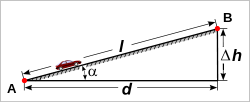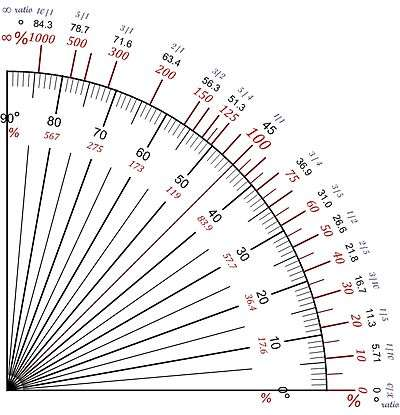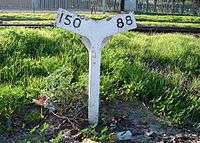d = run
Δh = rise
l = slope length
α = angle of inclination

The grade (also called slope, incline, gradient, mainfall, pitch or rise) of a physical feature, landform or constructed line refers to the tangent of the angle of that surface to the horizontal. It is a special case of the gradient in calculus where zero indicates gravitational level. A larger number indicates higher or steeper degree of "tilt". Often slope is calculated as a ratio of "rise" to "run", or as a fraction ("rise over run") in which run is the horizontal distance and rise is the vertical distance.

The grades or slopes of existing physical features such as canyons and hillsides, stream and river banks and beds are often described. Grades are typically specified for new linear constructions (such as roads, landscape grading, roof pitches, railroads, aqueducts, and pedestrian or bicycle circulation routes). The grade may refer to the longitudinal slope or the perpendicular cross slope.

## NomenclatureIllustration of grades (percentages), angles in degrees and ratio.

There are several ways to express slope:

1. as an angle of inclination to the horizontal. (This is the angle α opposite the "rise" side of a triangle with a right angle between vertical rise and horizontal run.)
2. as a percentage, the formula for which iswhich could also be expressed as the tangent of the angle of inclination times 100. In the U.S., this percentage "grade" is the most commonly used unit for communicating slopes in transportation (streets, roads, highways and rail tracks), surveying, construction, and civil engineering.
3. as a per mille figure, the formula for which iswhich could also be expressed as the tangent of the angle of inclination times 1000. This is commonly used in Europe to denote the incline of a railway.
4. as a ratio of one part rise to so many parts run. For example, a slope that has a rise of 5 feet for every 100 feet of run would have a slope ratio of 1 in 20. (The word "in" is normally used rather than the mathematical ratio notation of "1:20"). This is generally the method used to describe railway grades in Australia and the UK.

Any of these may be used. Grade is usually expressed as a percentage, but this is easily converted to the angle α from horizontal or the other expressions.

Slope may still be expressed when the horizontal run is not known: the rise can be divided by the hypotenuse (the slope length). This is not the usual way to specify slope; it follows the sine function rather than the tangent function, so it calls a 45-degree slope a 71-percent grade instead of a 100-percent. But in practice the usual way to calculate slope is to measure the distance along the slope and the vertical rise, and calculate the horizontal run from that. When the angle of inclination is small, using the slope length rather than the horizontal displacement (i.e., using the sine of the angle rather than the tangent) makes only an insignificant difference. Railway gradients are usually expressed in terms of the rise in relation to the distance along the track as a practical measure. In cases where the difference between sin and tan is significant, the tangent is used. In any case, the following identity holds for all inclinations up to 90 degrees:### Equations

Grades are related using the following equations with symbols from the figure at top.

Tangent as a ratioThis ratio can also be expressed as a percentage by multiplying by 100.If the tangent is expressed as a percentage, the angle can be determined as:If the angle is expressed as a ratio (1 in n) then:In vehicular engineering, various land-based designs (cars, SUVs, trucks, trains, etc.) are rated for their ability to ascend terrain. Trains typically rate much lower than cars. The highest grade a vehicle can ascend while maintaining a particular speed is sometimes termed that vehicle's "gradeability" (or, less often, "grade ability"). The lateral slopes of a highway geometry are sometimes called fills or cuts where these techniques have been used to create them.

The steepest roads in the world are Baldwin Street in Dunedin, New Zealand and Canton Avenue in Pittsburgh, Pennsylvania. The Guinness World Record lists Baldwin Street as the steepest street in the world, with a 35% grade (19°) overall and disputed 38% grade (21°) at its steepest section. 25000 balls of chocolate are rolled down the 350 m-long street in an annual charity Cadbury Jaffa Race. In 2001, a student was killed when the wheelie bin she rode down the street hit a trailer. The Pittsburgh Department of Engineering and Construction recorded a grade of 37% (20°) for Canton Avenue. The street has formed part of a bicycle race since 1983.

## Environmental design

Grade, pitch, and slope are important components in landscape design, garden design, landscape architecture, and architecture; for engineering and aesthetic design factors. Drainage, slope stability, circulation of people and vehicles, complying with building codes, and design integration are all aspects of slope considerations in environmental design.

## RailwaysGrade indicator near Bellville, Western Cape, South Africa, showing 1:150 and 1:88 grades.

Ruling gradients limit the load that a locomotive can haul, including the weight of the locomotive itself. On a 1% gradient (1 in 100) a locomotive can pull half (or less) of the load that it can pull on level track. (A heavily loaded train rolling at 20 km/h on heavy rail may require ten times the pull on a 1% upgrade that it does on the level at that speed.) Early railways in the United Kingdom were laid out with very gentle gradients, such as 0.05% (1 in 2000), because the early locomotives (and their brakes) were feeble. Steep gradients were concentrated in short sections of lines where it was convenient to employ assistant engines or cable haulage, such as the 1.2 kilometres (0.75 miles) section from Euston to Camden Town. Extremely steep gradients require the use of cables (such as the Scenic Railway at Katoomba Scenic World, Australia, with a maximum grade of 122% (52°), claimed to be the world's steepest passenger-carrying funicular) or some kind of rack railway (such as the Pilatus railway in Switzerland, with a maximum grade of 48% (26°), claimed to be the world's steepest rack railway) to help the train ascend or descend.

Gradients can be expressed as an angle, as feet per mile, feet per chain, 1 in n, x% or y per mille. Since surveyors like round figures, the method of expression can affect the gradients selected.

The steepest railway lines that do not use a rack system include:

### Compensation for curvature

Gradients on sharp curves are effectively a bit steeper than the same gradient on straight track, so to compensate for this and make the ruling grade uniform throughout, the gradient on those sharp curves should be reduced slightly.

### Continuous brakes

In the era before trains were provided with continuous brakes, whether air brakes or vacuum brakes, steep gradients were a serious problem, and it was difficult to stop safely if the line was on a steep grade. In an extreme example, the Inspector insisted that Rudgwick railway station in West Sussex be regraded before he would allow it to open. This required the gradient through the platform to be eased from 1 in 80 to 1 in 130.